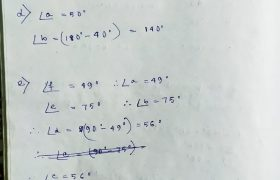## New Learning Composite Mathematics Class 7 SK Gupta Anubhuti Gangal Pairs and Angles Chapter 9F Solution

New Learning Composite Mathematics SK Gupta Anubhuti Gangal Class 7 Pairs and Angles Self Practice 9F Solution

## New Learning Composite Mathematics Class 8 SK Gupta Anubhuti Gangal Representing 3D Shapes in 2D Chapter 11 Solution

New Learning Composite Mathematics SK Gupta Anubhuti Gangal Class 8 Representing 3D Shapes in 2D Self Practice 11 Solution (9) (a) Answer: Sphere. (b) Answer: Cube. (c) Answer: Triangle Pyramid. (d) Answer: Rectangle Prism. (10) (a) i) Top View (ii) Front View (iii) Side View. (b) i) Side View ii) Front View iii) Top View. […]

## New Learning Composite Mathematics Class 7 SK Gupta Anubhuti Gangal Pairs and Angles Chapter 9E Solution

New Learning Composite Mathematics SK Gupta Anubhuti Gangal Class 7 Pairs and Angles Self Practice 9E Solution Self Practice 9A Solution Self Practice 9B Solution Self Practice 9C SolutionSK Gupta Anubhuti Gangal Solution Self Practice 9D SolutionSK Gupta Anubhuti Gangal Solution

## New Learning Composite Mathematics Class 7 SK Gupta Anubhuti Gangal Pairs and Angles Chapter 9D Solution

New Learning Composite Mathematics SK Gupta Anubhuti Gangal Class 7 Pairs and Angles Self Practice 9D Solution (1) Find each angle in the figure giving reasons. Self Practice 9A Solution Self Practice 9B Solution Self Practice 9C SolutionSK Gupta Anubhuti Gangal Solution

## New Learning Composite Mathematics Class 8 SK Gupta Anubhuti Gangal Quadrilaterals Chapter 10E Solution

New Learning Composite Mathematics SK Gupta Anubhuti Gangal Class 8 Quadrilaterals Self Practice 10E Solution (1) (a) ABCD in which AB = 4 cm, BC = 6 cm, CD = 5.5 cm, AD = 5 cm and AC = 8 cm. (b) PQRS in which PQ = 3.5 cm, QR = 5.5 cm, RS = […]

## New Learning Composite Mathematics Class 8 SK Gupta Anubhuti Gangal Quadrilaterals Chapter 10D Solution

New Learning Composite Mathematics SK Gupta Anubhuti Gangal Class 8 Quadrilaterals Self Practice 10D Solution Solution Number 1 to 7: (8) One of the diagonals of a rhombus is equal to one of its sides. Find the angles of the rhombus. Solution Number 8: (9) ABCD is a rectangle. E is he midpoint of AB. […]

## New Learning Composite Mathematics Class 8 SK Gupta Anubhuti Gangal Quadrilaterals Chapter 10C Solution

New Learning Composite Mathematics SK Gupta Anubhuti Gangal Class 8 Quadrilaterals Self Practice 10C Solution (1) Find the value of each variable in the parallelograms. Give brief reasons. (2) In the figure, AO = OD = OC and reflex <AOC = 2300. Find the value of x. Solution Number 1 & 2: (3) The measure […]

## New Learning Composite Mathematics Class 8 SK Gupta Anubhuti Gangal Quadrilaterals Chapter 10B Solution

New Learning Composite Mathematics SK Gupta Anubhuti Gangal Class 8 Quadrilaterals Self Practice 10B Solution (1) (a) A rectangle is a parallelogram Ans. Always (A). (b) A rhombus is a square Ans. Sometimes (S). (c) A parallelogram is a rhombus Ans. Sometimes (S). (d) A rhombus is a rectangle Ans. Sometimes (S). (e) A square […]

## New Learning Composite Mathematics Class 7 SK Gupta Anubhuti Gangal Pairs and Angles Chapter 9C SolutionNew Learning Composite Mathematics SK Gupta Anubhuti Gangal Class 7 Pairs and Angles Self Practice 9C Solution (1) Using property of corresponding angles, find each of the lettered angles giving reasons. (2) Using the properties of adjacent angles, vertically opposite angles and corresponding angles, fill in the size of each angle in diagram. Solution Number […]

## New Learning Composite Mathematics Class 8 SK Gupta Anubhuti Gangal Quadrilaterals Chapter 10A SolutionNew Learning Composite Mathematics SK Gupta Anubhuti Gangal Class 8 Quadrilaterals Self Practice 10A Solution (1) Find the value of unknown angle in each of the following figures. Solution Number 1: (2) Find the value of x. Solution Number 2: (3) Find the measures of the four angles of a quadrilateral if they are in […]